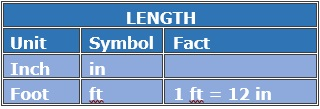# Conversions Involving Measurements in Feet and InchesConvert between feet(ft) and inches(in)

5 ft = __in

### Solution

Step 1:

1 ft = 12 in

Step 2:

So, 5 ft = 5 x 12 in = 60 in

Convert between feet(ft) and inches(in)

108 in = __ft

### Solution

Step 1:

1 ft = 12 in; 1 in = $\frac{1}{12}$ ft

Step 2:

So, 108 in = $\frac{108}{12}$ = 9 feet